# Midpoint 5

FM=3x-4, MG=5x-26, FG=? Point M is the midpoint of FG. Use the given information to find the missing measure or value.

m =  58

### Step-by-step explanation: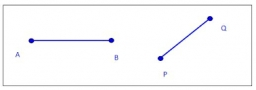Did you find an error or inaccuracy? Feel free to write us. Thank you!Tips to related online calculators
Do you have a linear equation or system of equations and looking for its solution? Or do you have a quadratic equation?
Do you want to convert length units?

## Related math problems and questions:

• Find midpoint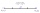FM=5y+13, MG=5-3y, FG=? M is the midpoint of FG. Use the given information to find the missing measure or value.
• Midpoint 6For line segment length is given: FM=8a+1, FG=42. Point M is the midpoint of FG. Find unknown a.
• MG=7x-15,Length of lines MG = 7x-15 and FG = 33 Point M is the midpoint of FG. Find the unknown x.
• The midpointThe midpoint of (2, 5) and (8, y) is (5, -1). Find the line equation in slope-intercept form.
• Line segmentThe 4 cm long line segment is enlarged in the ratio of 5/2. How many centimeters will measure the new line segment?
• Similar trianglesThe triangles ABC and XYZ are similar. Find the missing lengths of the sides of the triangles. a) a = 5 cm b = 8 cm x = 7.5 cm z = 9 cm b) a = 9 cm c = 12 cm y = 10 cm z = 8 cm c) b = 4 cm c = 8 cm x = 4.5 cm z = 6 cm
• Midpoint 4If the midpoint of a segment is (6,3) and the other end point is (8,-4) what are thw coordinate of the other end?
• Points OPQ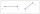Point P is on line segment OQ. Given OP = 6, OQ = 4x - 3, and PQ = 3x, find the numerical length of OQ.
• Midpoint of segmentPoint A has coordinates [4; -11] and the midpoint of the segment AB is the point [17; -7]. What are the coordinates of point B?
• SelectSelect the statement that describes this expression: 5 x (3 + 4) - 4. a) 5 times the sum of 3 and 4, then subtract 4 b) 5 times 3 plus 4 minus 4 c) 5 more than the sum of 3 and 4, and then subtract 4 d) 5 times 3 plus the difference of 3 and 4 What is the
• Coordinates of midpointIf the midpoint of the segment is (6,3) and the other end is (8,4) what are the coordinate of the other end?
• TranslateTranslate the following mathematical statement into an algebraic expression or equation. 1. Nine less that the quotient of a number and 3 2. The ratio of 3 and the sum of 4 and an unknown number 3. Eighty is the product of 4 and g 4. The sum of x and 18 i
• Line segmentFor the line segment whose endpoints are L[-1, 13] and M[18, 2], find the x and y value for the point located 4 over 7 the distance from L to M.
• Evaluate 9Evaluate expression: 3 { 4 − 4 ( 5 − 12 ) } + 20
• Median or middle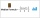The number of hours of television watched per day by a sample of 28 people is given below: 4, 1, 5, 5, 2, 5, 4, 4, 2, 3, 6, 8, 3, 5, 2, 0, 3, 5, 9, 4, 5, 2, 1, 3, 4, 7, 2, 9 What is the median value?
• Find the 5Find the equation of the circle with center at (1,20), which touches the line 8x+5y-19=0
• A cell tower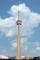A cell tower is located at coordinates (-5, -7) and has a circular range of 12 units. If Mr. XYZ is located at coordinates (4,5), will he be able to get a signal?